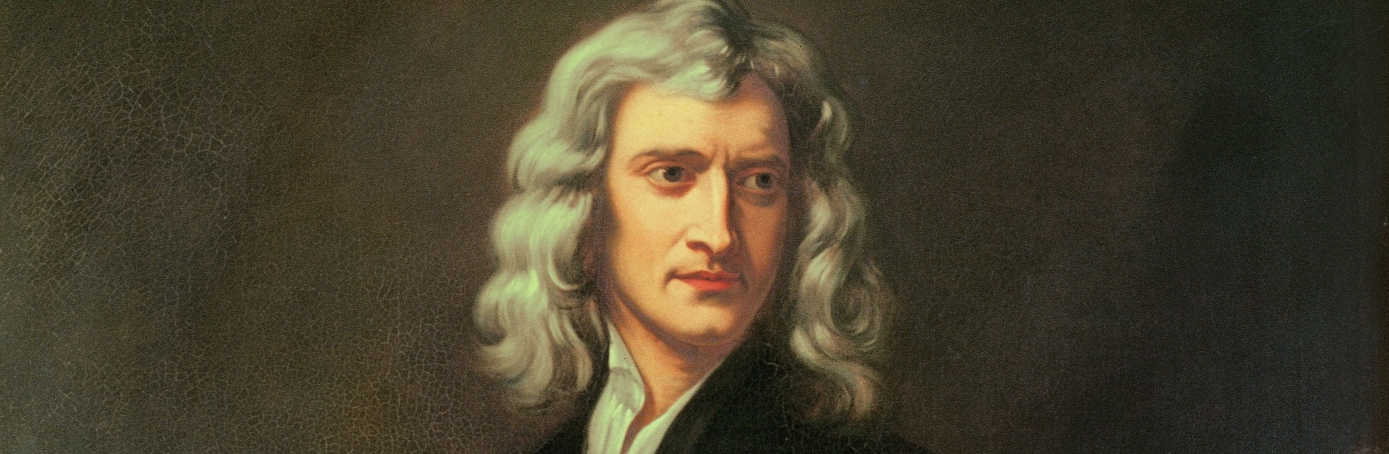# What are newton's three laws?

in Science
Need to know the three laws of newton
Every student will have gone through this in school times

## 1 Answer

by (225 points)

Newton commonly known as Issac Newton is a renowned Mathematician, astronomer and physicist Newton discovered calculus that enabled an easier way to solve problems in mathematics. ​image source: http://www.history.com/topics/isaac-newton

Newton also developed the three laws of motion that form the principles of basic physics. The three laws of Newton are mentioned below,

Newton’s First law

Every object in a state of uniform motion tends to remain in that state of motion unless an external force is applied to it

Newton’s Second law

The relationship between an object's mass m, its acceleration a, and the applied force F is F = ma. Acceleration and force are vectors (as indicated by their symbols being displayed in slant bold font); in this law, the direction of the force vector is the same as the direction of the acceleration vector

Newton’s Third Law

For every action there is an equal and opposite reaction